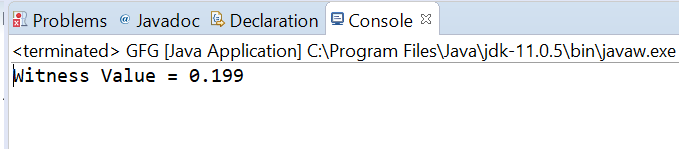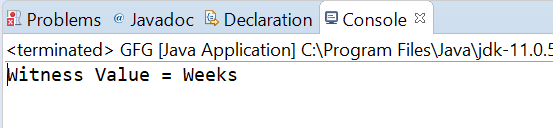# AtomicReference compareAndExchangeAcquire() method in Java with Examples

• Last Updated : 27 Dec, 2019

The compareAndExchangeAcquire() method of a AtomicReference class is used to Atomically sets the value to newValue to AtomicReference object, if the current value of AtomicReference object which is referred to as the witness value is equal to the expectedValue and returns the witness value. This method exchanges the value with memory ordering effects compatible with memory_order_acquire ordering.

Syntax:

```public final V compareAndExchangeAcquire(V expectedValue,
V newValue)
```

Parameters: This method accepts expectedValue which is the expected value and newValue which is the new value to set.

Return value: This method returns the witness value, which will be the same as the expected value if successful.

Below programs illustrate the compareAndExchangeAcquire() method:
Program 1:

 `// Java program to demonstrate``// AtomicReference.compareAndExchangeAcquire() method`` ` `import` `java.util.concurrent.atomic.AtomicReference;`` ` `public` `class` `GFG {``    ``public` `static` `void` `main(String[] args)``    ``{`` ` `        ``// create an atomic reference object``        ``// which stores Integer.``        ``AtomicReference ref``            ``= ``new` `AtomicReference();`` ` `        ``// set some value``        ``ref.set(``0.199``);`` ` `        ``// apply compareAndExchangeAcquire()``        ``double` `oldValue``            ``= ref.compareAndExchangeAcquire(``                ``0.199``,``                ``999.999``);`` ` `        ``// print value``        ``System.out.println(``"Witness Value = "``                           ``+ oldValue);``    ``}``}`

Output:Program 2:

 `// Java program to demonstrate``// AtomicReference.compareAndExchangeAcquire() method`` ` `import` `java.util.concurrent.atomic.AtomicReference;`` ` `public` `class` `GFG {``    ``public` `static` `void` `main(String[] args)``    ``{`` ` `        ``// create an atomic reference object.``        ``AtomicReference ref``            ``= ``new` `AtomicReference();`` ` `        ``// set some value``        ``ref.set(``"Weeks"``);`` ` `        ``// apply compareAndExchangeAcquire()``        ``String oldValue``            ``= ref.compareAndExchangeAcquire(``                ``"Weeks"``,``                ``"Days"``);`` ` `        ``// print value``        ``System.out.println(``"Witness Value = "``                           ``+ oldValue);``    ``}``}`

Output:My Personal Notes arrow_drop_up